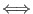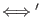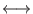Next: The ReadDRF keyword Up: CadenceTM Compatibility Previous: CadenceTM Compatibility   Contents   Index

## The Lisp Parser

The language supported here is similar to Lisp, and to the Cadence Skill language. The intention is not to replicate all features of these languages, but to provide a minimal subset of features for compatibility. The language will be referred to as Lisp'', but it should not be confused with the full-blown programming language.

The language differs from classic Lisp in that algebraic expressions within lists are evaluated, as in Skill. These reduce to a number token. One subtlety is detection of unary minus, for example (2 -1) could be interpreted as a list of two numbers, or one number (the difference). The parser will assume a unary minus if the preceding character is space or (', and the following character is an integer or period followed by an integer.

One of the advantages of Lisp is the ease with which the syntax can be parsed. The basic data object is a `node'', which has the form

[name]( data ... )
If a node has a name, there is no space between the name and the opening parenthesis. A named node is roughly equivalent to a function call. The data can be nodes, strings, or numerical expressions. The items are separated by white space. The data can use arbitrarily many lines in the input file.

Lisp variables are defined when assigned to, and have global scope unless declared in a let node, in which case their scope is within the let node, i.e., local.

A Lisp file consists of one or more named nodes. When the file is accessed with the !lisp command, each of the nodes is evaluated. The nodes must have names that are known to Xic. These are:

main
The content of this node is evaluated. This is a special name for the "main" function of a script.
Built-in function name
These are the basic Lisp functions and operator-equivalents.
Xic function name
All of the Xic script functions will be recognized, however in Lisp the first character of these functions is always lower case. i.e., the Edit script function would be accessed as edit( ) in Lisp. Also, only Xic functions that take string or numeric arguments will work at present.
User-defined procedures
These are Lisp functions defined by the user with the Lisp procedure( ) function.
There is a growing number of node names that are used to interpret Cadence startup and control files (see 5.7).

A node name that can't be resolved will generate an error.

The parser uses the same numerical parser as the WRspice program, and hence recognizes numbers in the same (SPICE) format. All of the math functions based on the standard C library, as used in the native scripting language, are available.

The following built-in node names are recognized.

 Operator Equivalents expt expt(x y)x^y times times(x y)x*y quotient quotient(x y)x/y plus plus(x y)x + y difference difference(x y)x - y lessp lessp(x y)x < y leqp leqp(x y)x < = y greaterp greaterp(x y)x > y geqp geqp(x y)x > = y equal equal(x y)x = = y nequal nequal(x y)x ! = y and and(x y)x && y or or(x y)x || y colon colon(x y)(xy)x : y setq setq(x y)x = y Lists ' returns list of arguments list returns substituted list of arguments cons add element to front of list append append lists car return leading element of list cdr return list starting at second element nth return N'th element of list member return true if element in list length return length of list xCoord return first element of list yCoord return second element of list Miscellaneous main main function procedure define a procedure argc command line argument count argv command line argument list let variable scope containerNext: The ReadDRF keyword Up: CadenceTM Compatibility Previous: CadenceTM Compatibility   Contents   Index
Stephen R. Whiteley 2019-03-16